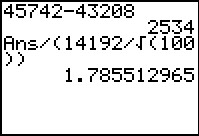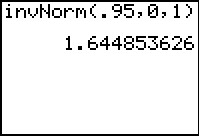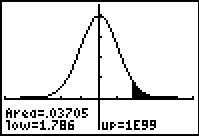••• ##### Device
• TI-83 Plus Family
• TI-84 Plus
• TI-84 Plus Silver Edition
•TI-84 Plus C Silver Edition
•TI-84 Plus CE
• ##### Report an Issue

Statistics: Hypothesis Testing: Means
by Texas Instruments#### Overview

Students test a claim about a mean with a large sample size at the five-percent significance level. The test statistic is found and compared to the critical value.

#### Key Steps

•In Problem 1, students are given a scenario to assist in testing a claim with a large sample size at five percent significance level.

The scenario describes the mean salary in a community, which an investor thinks is higher than the mean ten years ago. Students are asked to determine the null and alternative hypotheses.

They then decide whether to use the normal distribution or a t distribution for the test statistic and then calculate it.

•Students can find the critical value by using the invNorm command from the DISTR menu.

•Students will find the P-value using the ShadeNorm command, which finds the area under the curve to the right of the test statistic. From this value and comparing the test statistic to the critical value, students will decide whether to reject or fail to reject the null hypothesis.

At the end of this activity, students will be able to use the sample distribution of the mean of the population to test the null hypothesis against the alternative one-tailed hypothesis or two-tailed hypothesis.